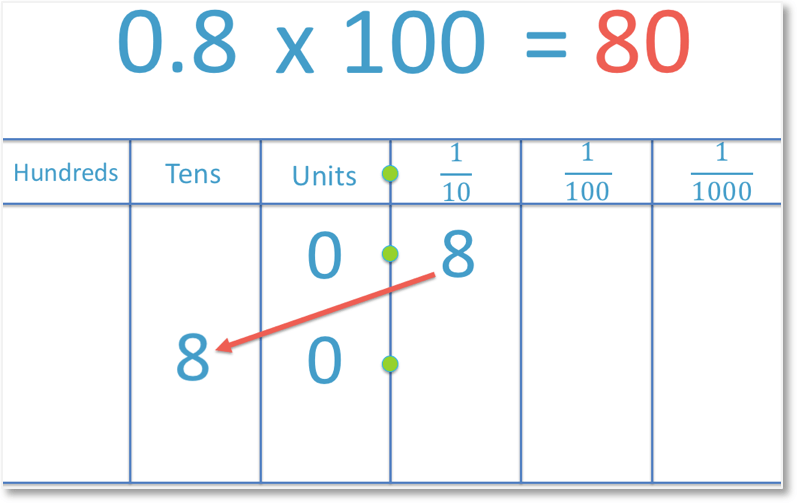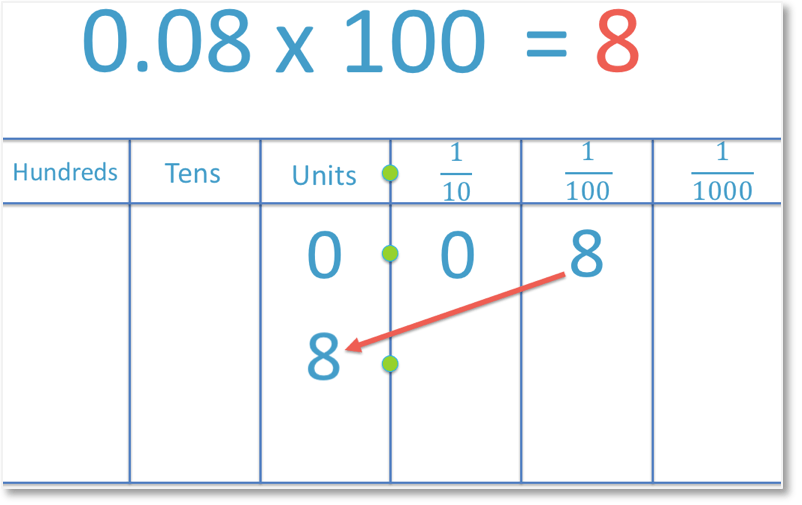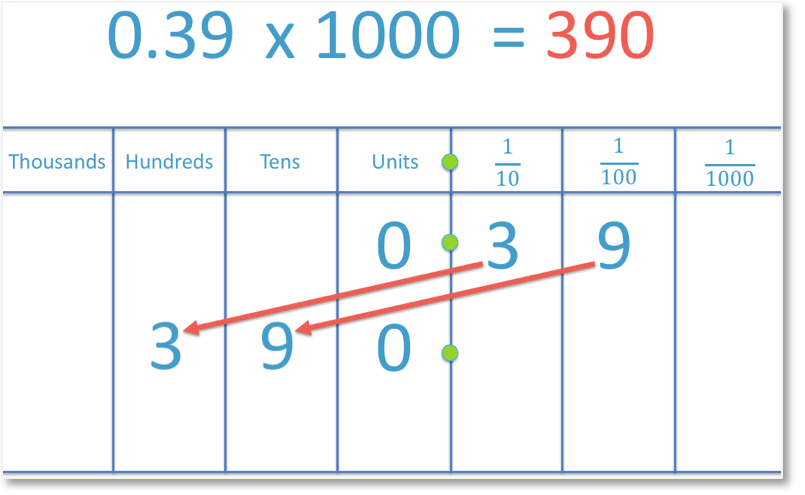# Multiplying by 100

Multiplying by 100• To multiply a number by one hundred, all of the digits in that number move two places to the left.
• We will multiply the number 8 by one hundred.
• The digit ‘8’ is in the units column and moves two places to the left into the hundreds column..
• There are no digits in the tens or units column and so, a zero is written in both of these columns.
• 8 x 100 = 800.
• Multiplying a whole number by one hundred is the same as adding two zero digits to this number.
To multiply a number by 100, move all of its digits two places to the left.

Multiplying a whole number by 100 is the same as adding two zero digits to the end of the number.• To multiply a number by 100, all digits of this number move two place value columns to the left.
• We will multiply the decimal number 5.2 by 100.
• The digit ‘5’ moves from the units column to the hundreds column.
• The digit ‘2’ moves from the tenths column to the tens column.
• There are no digits in the units column between the digit of ‘2’ and the decimal point and so a ‘0’ is put in its place.
• 5.2 x 100 = 520.Supporting Lessons# How to Multiply a Number by 100

When we multiply a number by 100 we move each digit in that number two place value columns to the left.

Multiplying a whole number by 100 is the same as adding two zeros to the end of the number.

In the example below we will multiply the whole number 8 by one hundred.In the example above, the 8 has been moved two places to the left. It has moved from the units column to the hundreds column. As there are no longer any digits in either the tens column or the units column, we write a zero in both spaces to show that they are worth zero.

8 is a whole number with no digits after the decimal point. Therefore we can use the trick of “adding two zeros” to the end of the number. This trick only works when multiplying whole numbers by one hundred.

It is important to understand how multiplying by 100 works because not all numbers that we will be multiplying will be whole numbers.

Here is an example of multiplying a decimal number by 100:To multiply the decimal 0.8 by 100, we move the 8 two places to the left. We move it from the tenths column to the tens column. We must also make sure that we show that the units column is worth zero by writing a zero in that space.

And so, 0.8 x 100 = 80.

In the following example, we are asked to multiply the decimal 0.08 by 100.To do this, we move the 8 two places to the left, from the hundredths column to the units column. And so, 0.08 x 100 = 8

When multiplying a number with more than one digit by 100, we need to make sure that we move every digit two places to the left.

For example:To multiply 5.2 by 100, we begin by moving the 5 two places to the left. It moves from the units column to the hundreds column. The 2 can then follow. We move the 2 from the tenths column to the tens column.

Because there are no longer any digits in the units column, we must write in a zero to show that the column is worth zero. Therefore, 5.2 x 100 = 520.

We need to be more careful when dealing with numbers where there is a zero between two other digits.

For example:We must make sure that we move every digit in 3.901 two places to the left, including the zero.

We move the 3 from the units column to the hundreds column, we move the 9 from the tenths column to the tens column, we move the 0 from the hundredths column to the units column and we move the 1 from the thousandths column to the tenths column.

Therefore, 3.901 x 100 = 390.1.Now try our lesson on Multiplying by 1000 where we learn how to multiply a number by 1000.